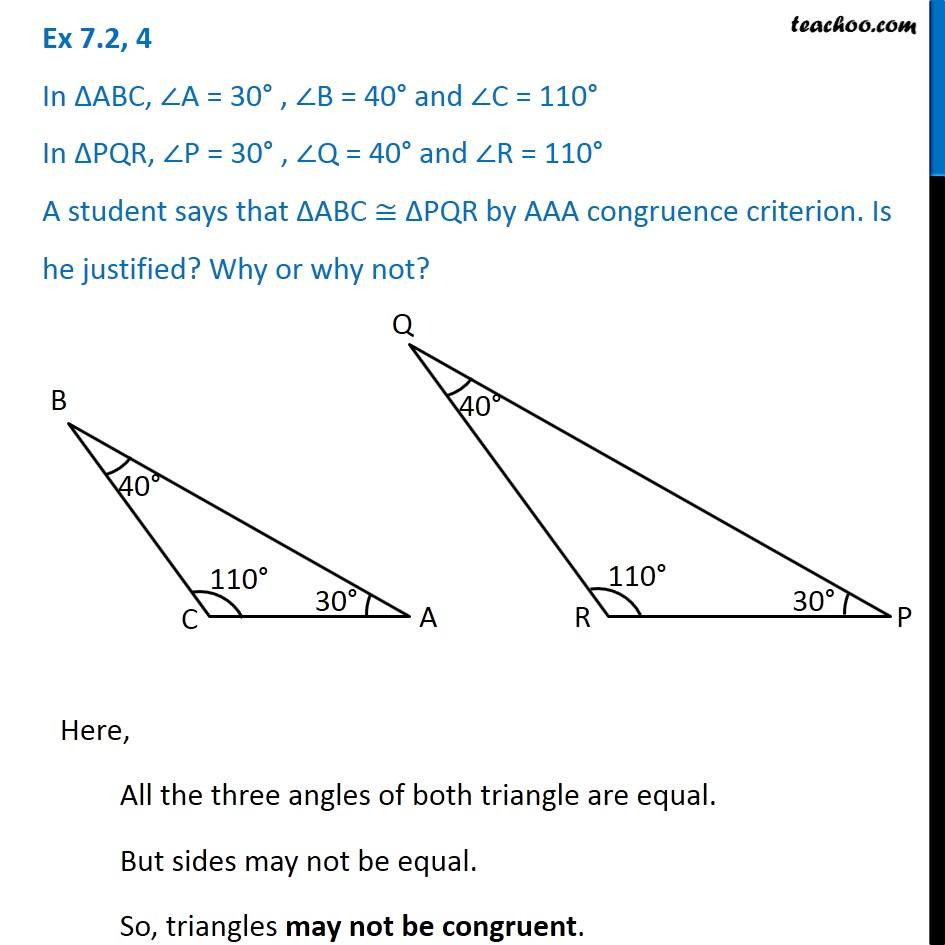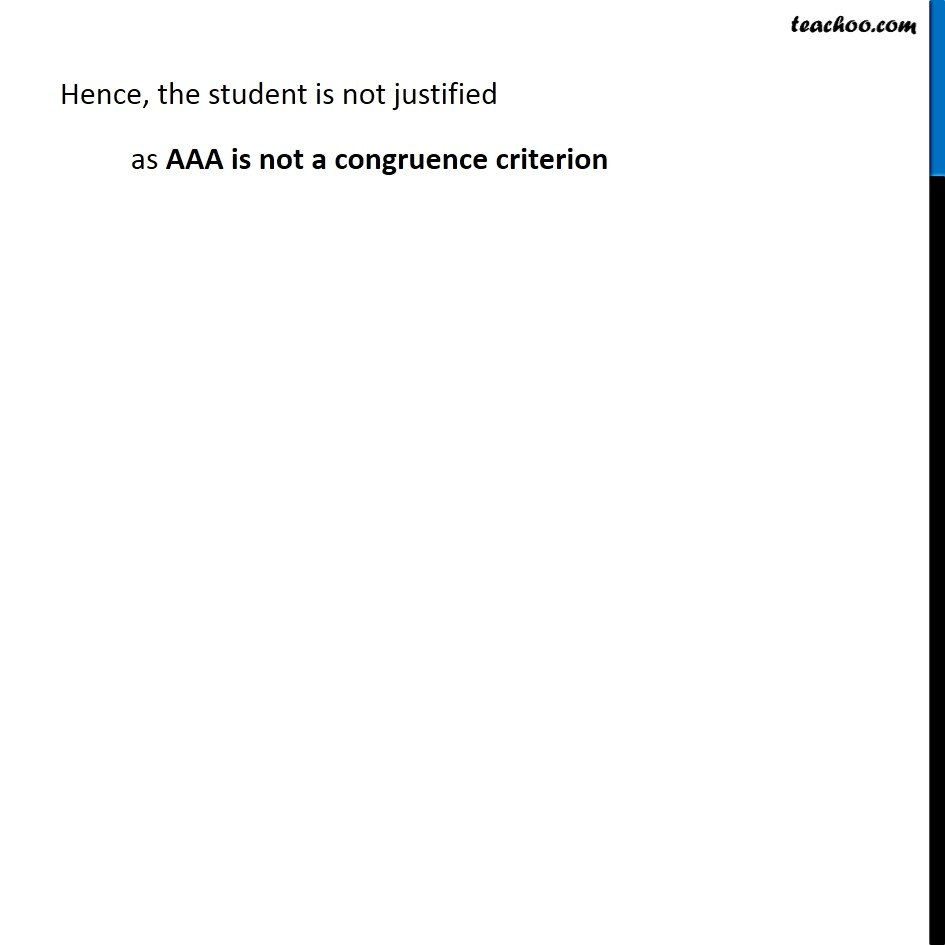1. Chapter 7 Class 7 Congruence of Triangles
2. Serial order wise
3. Ex 7.2

Transcript

Ex 7.2, 4 In ∆ABC, ∠A = 30° , ∠B = 40° and ∠C = 110° In ∆PQR, ∠P = 30° , ∠Q = 40° and ∠R = 110° A student says that ∆ABC ≅ ∆PQR by AAA congruence criterion. Is he justified? Why or why not? Here, All the three angles of both triangle are equal. But sides may not be equal. So, triangles may not be congruent. Hence, the student is not justified as AAA is not a congruence criterion